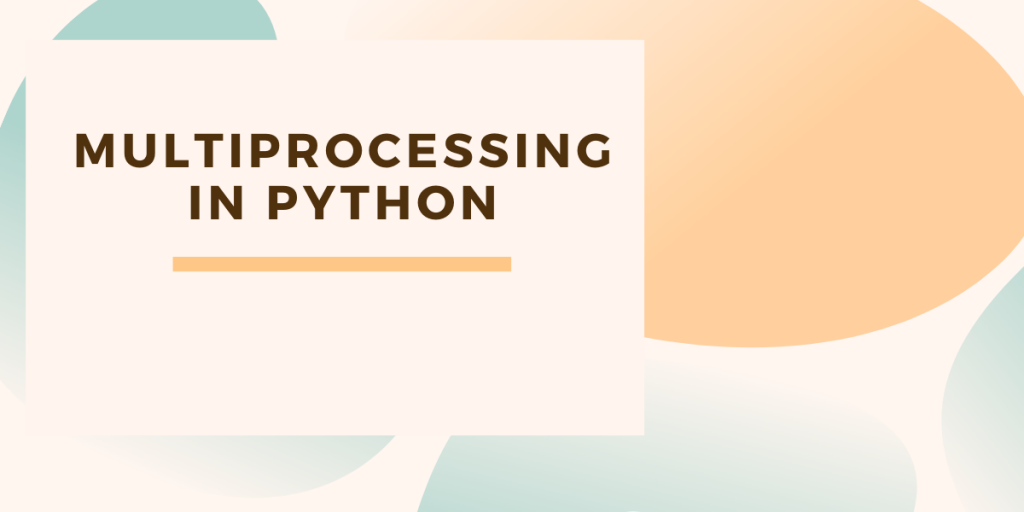# Multiprocessing In Python## What is multiprocessing?

Multiprocessing is a package in python that supports the ability to spawn processes that make use of a Python API. It similar to the threading module in Python.

## Understanding Multiprocessing in Python

A multiprocessor is a computer means that the computer has more than one central processor. If a computer has only one processor with multiple cores, the tasks can be run parallel using multithreading in Python.

A multiprocessor system has the ability to support more than one processor at the same time. To find the number of CPU cores available on our system, we use mp.cpu_count() function.

Here’s a sample code to find processor count in Python using the multiprocessing module:

```import multiprocessing as mp

print(mp.cpu_count())
```

Output: 12

The count here is the total number of cores between multiple processors, summed up.

The four most important classes of this module are-

• Process Class
• Lock Class
• Queue Class
• Pool Class

Let’s look at each of these classes individually…

### 1. Process Class

Process is the forked copy of the current process. It creates a new process identifier and tasks run as independent child process.

start() and join() functions belong to this class. To pass an argument through a process, we use args keyword.

Example of start() function-

Here, we have created a function calc_square and calc_cube for finding square and cube of the number respectively. In the main function we have created the objects p1 and p2. p1.start() and p2.start() will start the function and calling p1.join() and p2.join will terminate the process.

```import time
import multiprocessing

def calc_square(numbers):
for n in numbers:
print('square ' + str(n*n))

def calc_cube(numbers):
for n in numbers:
print('cube '+ str(n*n*n))

if __name__ == "__main__":
arr=[2,3,8,9]
p1=multiprocessing.Process(target=calc_square,args=(arr,))
p2=multiprocessing.Process(target=calc_cube,args=(arr,))

p1.start()
p2.start()

p1.join()
p2.join()

print("Done")
```

Output:

```square 4
square 9
square 64
square 81
cube 8
cube 27
cube 512
cube 729
Done
```

### 2. Lock Class

The lock class allows the code to be locked in order to make sure that no other process can execute the similar code until it is released.

To claim the lock, acquire() function is used and to release the lock, release() function is used.

```from multiprocessing import Process, Lock

lock=Lock()
def printer(data):
lock.acquire()
try:
print(data)
finally:
lock.release()

if __name__=="__main__":
items=['mobile','computer','tablet']
for item in items:
p=Process(target=printer,args=(item,))
p.start()
```

Output

```mobile
computer
tablet
```

### 3. Queue Class

Queue is a data structure which uses First In First Out (FIFO) technique.It helps us perform inter process communication using native Python objects.

Queue enables the Process to consume shared data when passed as a parameter.

put() function is used to insert data to the queue and get() function is used to consume data from the queue.

```import multiprocessing as mp

def sqr(x,q):
q.put(x*x)

if __name__ == "__main__":
q=mp.Queue() # Instance of queue class created
processes=[mp.Process(target=sqr,args=(i,q))for i in range (2,10)] # List of processes within range 2 to 10
for p in processes:
p.start()

for p in processes:
p.join()

result = [q.get() for p in processes]
print(result)
```

Output:

```[4, 9, 16, 25, 36, 64, 49, 81]
```

### 4. Pool Class

The pool class helps us execute a function against multiple input values in parallel. This concept is called Data Parallelism.

Here, array [5,9,8] is mapped as input in the function call. pool.map() function is used to pass a list of multiple arguments.

```import multiprocessing as mp

def my_func(x):
print(x**x)

def main():
pool = mp.Pool(mp.cpu_count())
result = pool.map(my_func, [5,9,8])

if __name__ == "__main__":
main()
```

Output:

```3125
387420489
16777216
```

## Conclusion

In this article, we learned the four most important classes in multiprocessing in Python – Process, Lock, Queue, and Pool which enables better utilization of CPU cores and improves performance.

## References

Official Module documentation